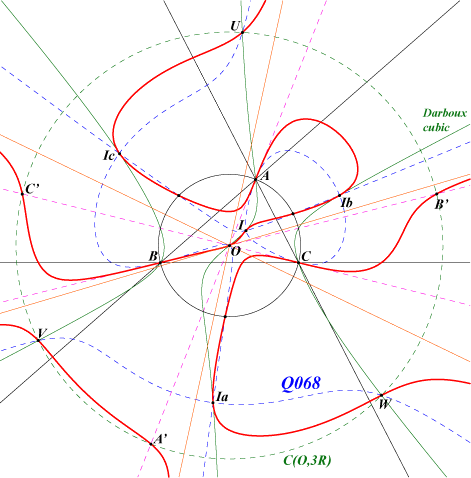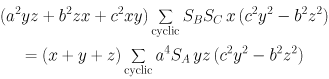X(1), X(3) excenters common points of the circumcircle and the Thomson cubic infinite points of the Orthocubic three points U, V, W on C(O,3R) and on the Darboux cubic images of A, B, C under h(O,–3) also on C(O,3R)Let k be a real number, P a point and P* its isogonal conjugate. Consider the triangles : – P(k) homothetic of the pedal triangle of P under the homothety with center P, ratio k, – T(k) homothetic of ABC under the homothety with center P*, ratio k. These triangles P(k) and T(k) are orthologic and the centers of orthology are P and P*. They are perspective if and only if P lies on a circular quintic Q'(k). We have Q'(2) = Q067 and Q'(1) is the union of the circumcircle and the Darboux cubic. The limit case Q'(k->0) is Q068 and the limit case Q'(k->∞) is Q069. Q069 is a circular quintic with singular focus O. It has three real asymptotes parallel to those of the Orthocubic and also concurring at O. In other words, the polar lines of the five infinite points of Q069 pass through O. The locus of the finite points having a polar line with respect to Q069 passing through O is a pK with isopivot O. In particular, the tangents at A, B, C to Q069 pass through O and A, B, C are inflexion points on Q069. The tangents at the in/excenters pass through X(20) hence Q069 is tangent to the Darboux cubic at these points. Q069 meets : – the circumcircle at the same points as the Thomson cubic. – the circle C(O, 3R) at three points U, V, W described in the Darboux cubic page and three other points A', B', C' images of A, B, C under h(O,–3).